﻿PrintImages Method (Int32, Int32, Double, Double, Boolean, Double, Double, Double, Double, Boolean, Boolean, Boolean)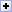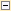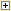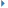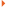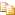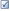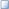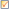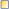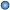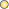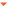PrintImages prints all images or only selected images.

You can specify number of columns and rows, spaces between images and margins. It is possible also to draw box around images, shadows and text.

Namespace: HiComponents.IEvolution
Assembly: IEvolution2 (in IEvolution2.dll) Version: 10.0.0.0

#Syntax

 C# Visual Basic Visual C++
```public void PrintImages(
int columns,
int rows,
double horizSpace,
double vertSpace,
bool printSelected,
double marginLeft,
double marginTop,
double marginRight,
double marginBottom,
bool drawBox,
bool drawText,
)```
```Public Sub PrintImages ( _
columns As Integer, _
rows As Integer, _
horizSpace As Double, _
vertSpace As Double, _
printSelected As Boolean, _
marginLeft As Double, _
marginTop As Double, _
marginRight As Double, _
marginBottom As Double, _
drawBox As Boolean, _
drawText As Boolean, _
)```
```public:
void PrintImages(
int columns,
int rows,
double horizSpace,
double vertSpace,
bool printSelected,
double marginLeft,
double marginTop,
double marginRight,
double marginBottom,
bool drawBox,
bool drawText,
)```

#### Parameters

columns
Int32
Specifies how arrange images, specifying the number of columns.
rows
Int32
Specifies how arrange images, specifying the number of rows.
horizSpace
Double
Horizontal space in inches between images
vertSpace
Double
Vertical space in inches between images
printSelected
Boolean
True if you want to print only selected images
marginLeft
Double
Page left margin in inches. By specifying zero, no margin is used
marginTop
Double
Page top margin in inches. By specifying zero, no margin is used
marginRight
Double
Page right margin in inches. By specifying zero, no margin is used
marginBottom
Double
Page bottom margin in inches. By specifying zero, no margin is used
drawBox
Boolean
True if you want to draw a box around the images (image space). Image is always stretched to maintain aspect ratio.
drawText
Boolean
True if you want to draw text associated with every image